# Aggregate Points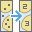The Aggregate Points tool uses area features to summarize a set of point features. The boundaries from the area feature are used to collect the points within each area and use them to calculate statistics. The resulting layer displays the count of points within each area.

## Analysis using GeoAnalytics Tools

Analysis using GeoAnalytics Tools is run using distributed processing across multiple ArcGIS GeoAnalytics Server machines and cores. GeoAnalytics Tools and standard feature analysis tools in ArcGIS Enterprise have different parameters and capabilities. To learn more about these differences, see Feature analysis tool differences.

## Examples

##### Tip:

If your portal is configured to use Living Atlas content, you can use the state and county Living Atlas layers, which include population data.

## Usage notes

Aggregate Points is designed to collect and summarize point features within a set of boundaries. The input parameters must include points to be aggregated and aggregation areas.

You can provide the area layer to use for analysis, or you can generate bins of a specified size and shape (hexagon or square) into which to aggregate. The bin size specifies how large the bins are. If you are aggregating into hexagons, the size is the height of each hexagon and the width of the resulting hexagon will be 2 times the height divided by the square root of 3. If you are aggregating into squares, the bin size is the height of the square, which is equal to the width.

Only areas that contain points will be returned, and resulting areas will be completely removed from the result layer.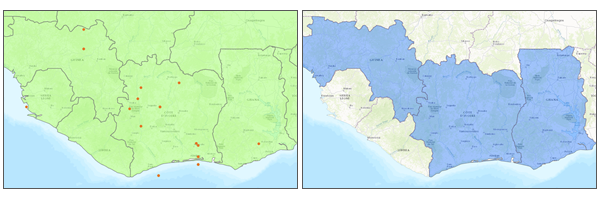The input point and area features (left), and the resulting area features (right).

The most basic aggregations will calculate a count of the number of points in each boundary. Statistics (count, sum, minimum, maximum, range, mean, standard deviation, and variance) can also be calculated on numerical fields, and statistics (count, any) can be calculated on string fields. The statistics will be calculated on each area separately.

##### Note:

When count is applied to a field, it returns a count of the nonnull values present in the field. When any is applied to a string field, it returns a single string present in the field.

Aggregate Points allows you to optionally analyze using time steps. Each time step is analyzed independently of features outside of the time step. To use time stepping, your input data must be time-enabled and represent an instant in time. When time stepping is applied, output features will be time intervals represented by the fields START_DATETIME and END_DATETIME.

Aggregate Points requires that your area layer be in a projected coordinate system. If your data is not in a projected coordinate system and you do not set a projected processing coordinate system, a projection will be picked based on the extent of the data you are analyzing.

If Use current map extent is checked, only the features that are visible within the current map extent will be analyzed. If it's not checked, all input features in the input layer will be analyzed, even if they are outside the current map extent.

## Limitations

Inputs must include a point layer. The area to aggregate into must be a provided area layer or bins. Lines and areas cannot be aggregated into areas using the Aggregate Points tool.

## How Aggregate Points works

### Equations

Variance is calculated using the following equation: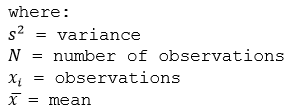Standard deviation is calculated as the square root of the variance.

### Calculations

Point layers are summarized using only the point features intersecting the input boundary. The results are displayed in blue and can be symbolized using graduated symbols on the calculated statistics.

The following figure and table illustrate the statistical calculations of a point layer within district boundaries. The Population field was used to calculate the statistics (Count,Sum, Minimum, Maximum, Range, Mean, Standard Deviation, and Variance) for the layer. The Type field was used to calculate the statistics (Count and Any) for the layer.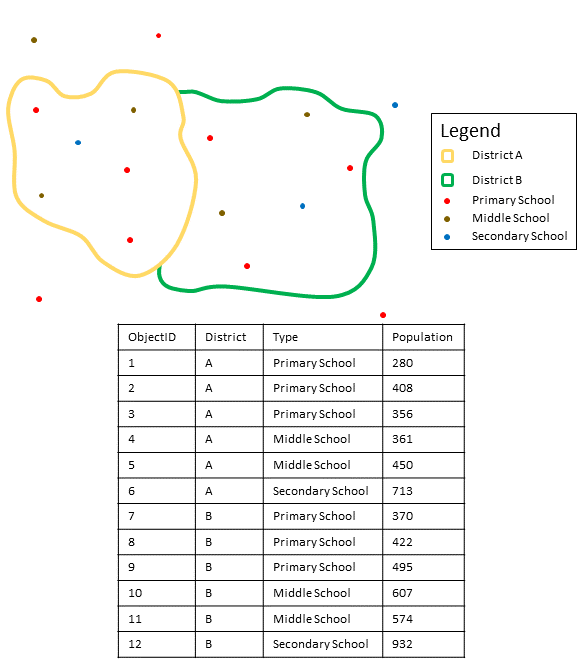Point layers are summarized using only points located within the boundary layer. An example attribute table is displayed above with values to be used in this hypothetical statistical calculation.
Numeric statisticResults District A

Count

Count of:

``[280, 408, 356, 361, 450, 713] = 6``

Sum

``280 + 408 + 356 + 361 + 450 + 713 = 2568``

Minimum

Minimum of:

``[280, 408, 356, 361, 450, 713] = 280``

Maximum

Maximum of:

``[280, 408, 356, 361, 450, 713] = 713``

Average

``2,568/6 = 428``

Variance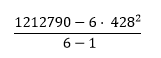``= 22737.2``

Standard Deviation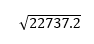``= 150.7886``
String statisticResults District A

Count

``= 6``

Any

= Secondary School

The count statistic (for strings and numeric fields) counts the number of nonnull values. The count of the following values equals 5: [0, 1, 10, 5, null, 6] = 5. The count of this set of values equals 3: [Primary, Primary, Secondary, null] = 3.

A real-life scenario in which this analysis could be used is in determining the total number of students in each school district. Each point represents a school. The Type field gives the type of school (elementary, middle school, or secondary), and a student population field gives the number of students enrolled at each school. The calculations and results are given for District A in the table above. From the results, you can see that District A has 2,568 students. When running the Aggregate Points tool, the results would also be given for District B.

## ArcGIS API for Python example

The Aggregate Points tool is available through ArcGIS API for Python.

This example aggregates a crime dataset into an area layer of census tracts. It calculates the sum of officers on site for each polygon.

``````# Import the required ArcGIS API for Python modules
import arcgis
from arcgis.gis import GIS
from arcgis.geoanalytics import summarize_data

# Connect to your ArcGIS Enterprise portal and check that GeoAnalytics is supported
portal = GIS("https://myportal.domain.com/portal", "gis_publisher", "my_password", verify_cert=False)

if not portal.geoanalytics.is_supported():
print("Quitting, GeoAnalytics is not supported")
exit(1)

# Find the big data file share dataset you're interested in using for analysis
search_result = portal.content.search("", "Big Data File Share")

# Look through search results for a big data file share with the matching name
bd_file = next(x for x in search_result if x.title == "bigDataFileShares_myBigDataFileShare")

# Look through the big data file share for a dataset called Crimes
crime_data = next(x for x in bd_file.layers if x.properties.name == "Crimes")

# Find a feature layer named "Census_Tracts" in your ArcGIS Enterprise portal
census_tract = portal.content.search("Census_Tracts", "Feature Layer")
census_tract_layer = census_tract_search_result.layers

# Set the environment settings for this tool run
arcgis.env.verbose = True

# Run the tool
crime_data_aggregate = summarize_data.aggregate_points(point_layer = crime_data,
polygon_layer = census_tract_layer,
summary_fields = [{'statisticType' : 'Sum',
'onStatisticField' : 'OfficersOnSite'}],
output_name = 'Crime_Data_Aggregated')

# Visualize the tool results if you are running Python in a Jupyter Notebook
processed_map = portal.map('MyCity, State', 10)
processed_map``````

## Similar tools

Use Aggregate Points to summarize points within areas. Other tools may be useful in solving similar but slightly different problems.

### Map Viewer analysis tools

If you are trying to summarize lines or areas into areas or bins, use the GeoAnalytics Tools .

If you are trying to summarize points, lines, or areas using different spatial relationships, use the GeoAnalytics Tools Join Features.

If you are trying to summarize lines or areas, use the standard tool Summarize Within.

If you would like to aggregate points into areas using the standard analysis tools, see Aggregate Points.

### ArcGIS Desktop analysis tools

The GeoAnalytics Tools Aggregate Points is available in ArcGIS Pro.

Aggregate Points performs the functions of the Spatial Join and Summary Statistics tools.